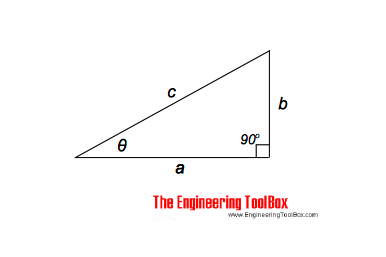Engineering ToolBox - Resources, Tools and Basic Information for Engineering and Design of Technical Applications!

# Right Angled Triangle

## Right angled triangle equations.

A right triangle - or a right angled triangle - is a triangle in which one of the angles is a right angle - a 90 degree angle.The length of the hypotenuse of aright triangle can be calculated as

c = (a2 + b2)1/2                                     (1)

The other sides can be calculated as

a = (c2 - b2)1/2                                     (2)

b = (c2 - a2)1/2                                     (3)

The angle θ can be calculated as

θ = atan b/a

= arctan b/a

= tan-1 b/a                                       (4)

### Example - Right Triangle

The length of the hypotenuse where a = 1.7 m and b = 1 m can be calculated as

c = ((1.7 m)2 + (1 m)2)1/2

= 1.97 m

The angle θ can be calculated as

θ = atan ((1 m) / (1 m))

= 30.5o

## Related Topics

• ### Mathematics

Mathematical rules and laws - numbers, areas, volumes, exponents, trigonometric functions and more.

## Related Documents

• ### Angle Calculator - Carpenter's Square

Calculate angles with a straight board across carpenter's square.
• ### Center of Gravity

A body and the center of gravity.
• ### Centroids of Plane Areas

The controid of square, rectangle, circle, semi-circle and right-angled triangle.
• ### Geometric Shapes - Areas

Areas, diagonals and more - of geometric figures like rectangles, triangles, trapezoids ..
• ### Law of Cosines

One side of a triangle when the opposite angle and two sides are known.
• ### Law of Sines

Calculate the angles in a generic triangle.
• ### Law of Tangents

Triangles and law of tangents.
• ### Miter Saw - Calculate Sawing Angle

Calculate miter saw protractor angles for skirting and decorative mouldings work.
• ### Oblique Triangle

Calculate oblique triangles.
• ### Pythagorean Theorem

Verifying square corners.
• ### Regular Polygons

Areas of regular polygons - polygons with 3 to 12 sides.
• ### Triangle

Triangle analytical geometry.
• ### Trigonometric Functions

Sine, cosine and tangent - the natural trigonometric functions.

Online vector calculator - add vectors with different magnitude and direction - like forces, velocities and more.

## Engineering ToolBox - SketchUp Extension - Online 3D modeling!

Add standard and customized parametric components - like flange beams, lumbers, piping, stairs and more - to your Sketchup model with the Engineering ToolBox - SketchUp Extension - enabled for use with older versions of the amazing SketchUp Make and the newer "up to date" SketchUp Pro . Add the Engineering ToolBox extension to your SketchUp Make/Pro from the Extension Warehouse !

We don't collect information from our users. More about

## Citation

• The Engineering ToolBox (2013). Right Angled Triangle. [online] Available at: https://www.engineeringtoolbox.com/right-triangle-d_1859.html [Accessed Day Month Year].

Modify the access date according your visit.

10.2.10